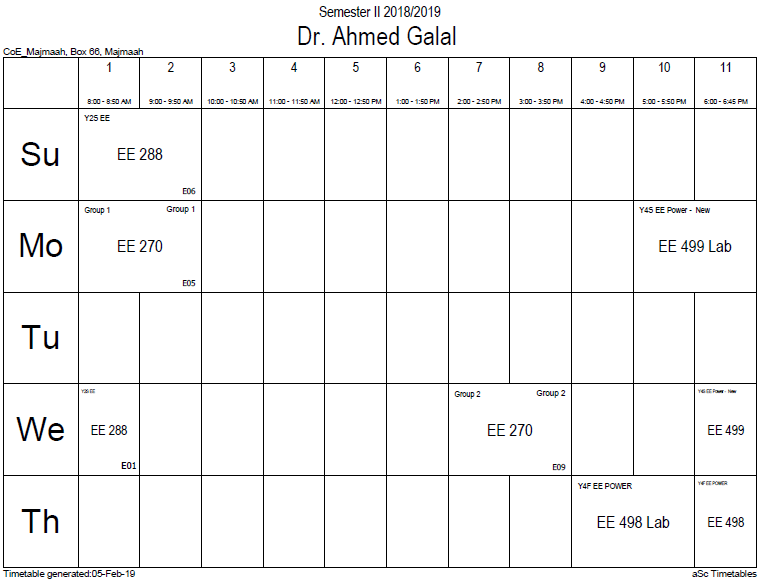## Convolutionsgroups

If G is a suitable group endowed with a measure λ, and if f and g are real or complex valued integrable functions on G, then we can define their convolution by$(f * g)(x) = int_G f(y) g(y^{-1}x),dlambda(y). ,$

In typical cases of interest G is a locally compact Hausdorff topological group and λ is a (left-) Haar measure. In that case, unless G is unimodular, the convolution defined in this way is not the same as$extstyle{int f(xy^{-1})g(y) , dlambda(y)}$. The preference of one over the other is made so that convolution with a fixed function g commutes with left translation in the group:$L_h(f*g) = (L_hf)*g = f*(L_hg).,$

Furthermore, the convention is also required for consistency with the definition of the convolution of measures given below. However, with a right instead of a left Haar measure, the latter integral is preferred over the former.

On locally compact abelian groups, a version of the convolution theorem holds: the Fourier transform of a convolution is the pointwise product of the Fourier transforms. The circle group T with the Lebesgue measure is an immediate example. For a fixed g in L1(T), we have the following familiar operator acting on the Hilbert space L2(T):$T {f}(x) = frac{1}{2 pi} int_{mathbf{T}} {f}(y) g( x - y) , dy.$

The operator T is compact. A direct calculation shows that its adjoint T* is convolution with$ar{g}(-y). ,$

By the commutativity property cited above, T is normal: T*T = TT*. Also, T commutes with the translation operators. Consider the family S of operators consisting of all such convolutions and the translation operators. Then S is a commuting family of normal operators. According to spectral theory, there exists an orthonormal basis {hk} that simultaneously diagonalizes S. This characterizes convolutions on the circle. Specifically, we have$h_k (x) = e^{ikx}, quad k in mathbb{Z},;$

which are precisely the characters of T. Each convolution is a compact multiplication operator in this basis. This can be viewed as a version of the convolution theorem discussed above.

A discrete example is a finite cyclic group of order n. Convolution operators are here represented by circulant matrices, and can be diagonalized by the discrete Fourier transform.

A similar result holds for compact groups (not necessarily abelian): the matrix coefficients of finite-dimensional unitary representations form an orthonormal basis in L2 by the Peter–Weyl theorem, and an analog of the convolution theorem continues to hold, along with many other aspects of harmonic analysis that depend on the Fourier transform.

### Office HoursNo office hours### My Timetable### Contactsemail: [email protected]

Phone: 2570

### Welcome

Welcome To Faculty of Engineering### IEEEhttp://www.ieee.org/

/

### Bookmarkshttp://www.utk.edu/research/

http://science.doe.gov/grants/index.asp

http://www1.eere.energy.gov/vehiclesandfuels/

http://www.eere.energy.gov/

### Upcoming Conferences### Engineering quotes### Travel Web Siteshttp://www.hotels.com/

http://www.orbitz.com/

http://www.hotwire.com/us/index.jsp

http://www.kayak.com/

### Blackboardستقام اختبارات الميدتيرم يوم الثلاثاء 26-6-1440

حسب الجدول المعلن بلوحات الاعلان

### Summer trainingThe registration for summer training will start from 5th week of second semesterClass registration week 1

### برنامج التجسير### إحصائية الموقع

عدد الصفحات: 2879

البحوث والمحاضرات: 1280

الزيارات: 100898